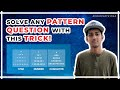ARTICLEViews: 29Posted by - Anonymous

# Tips to solve the DSA questions by recognizing their pattern.

Tips to solve the DSA questions by recognizing their pattern.

If given a linked list then

✒ Two pointers

If the input array is sorted then

✒ Binary search

✒ Two pointers

If asked for all permutations/subsets then

✒ Backtracking

If given a tree or a graph then

✒ DFS

✒ BFS

If recursion is banned then

✒ Stack

If must solve in-place then

✒ Swap corresponding values

✒ Store one or more different values in the same pointer

If asked for maximum/minimum subarray/subset/options then

✒ Dynamic programming

If asked for top/least K items then

✒ Heap

If asked for common strings then

✒ Map

✒ Trie

General Tips

✒ Map/Set for O(1) time & O(n) space

✒ Sort input for O(nlogn) time and O(1) space

How to solve 500 Data Structures and Algorithms Question. This strategy for Coding style will allow you to solve maximum ...Solve Any Pattern Question with This Trick!Solve ANY Problem on Leetcode | Most Common Leetcode DSA PatternsSolve DSA questions using this trick! #shortsHow to Identify Patterns in DSA Problems? | 6 Type of Interval Problems Asked in FAANG Interviews

Views -